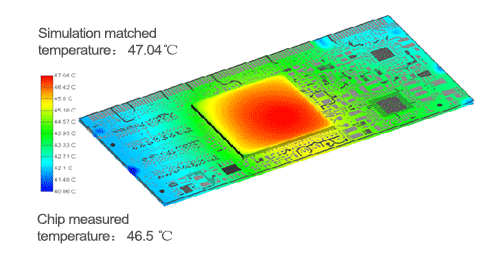When is Power Integrity (PI) Simulation Required?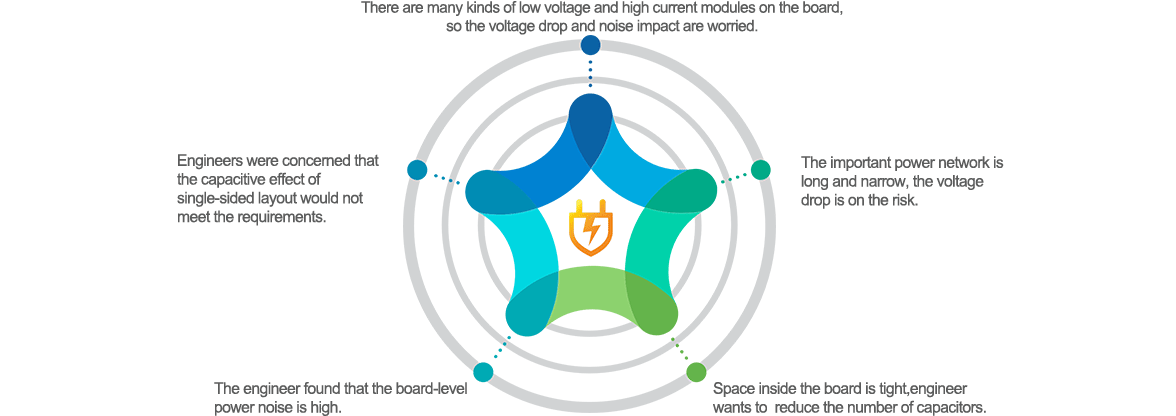1

Product diversification

Rich engineering experience

2

Simulation and test comparison

Continuously improve simulation accuracy

3

Through simulation and accurate power supply

Test to help solve power problems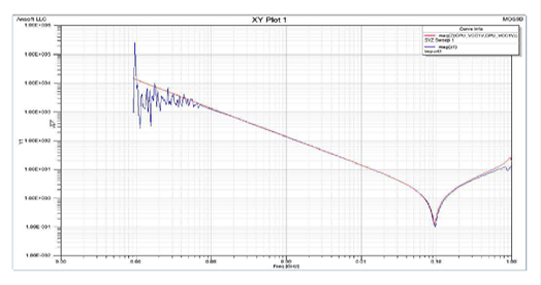Power network impedance simulation test comparison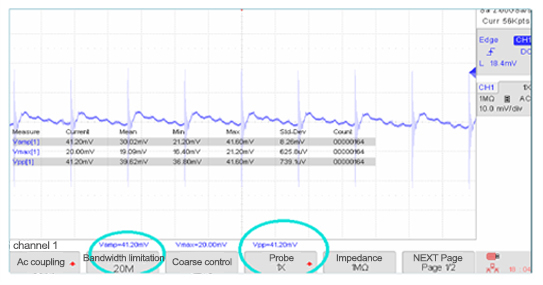Power noise for actual test

What is the Content of Power Integrity (PI) Simulation?

IR-Drop Simulation

-Determine whether the power voltage drop meets the requirements

-Simulation and analysis of current density, current carrying capacity, etc.

-Simulation analysis of optimal feedback point position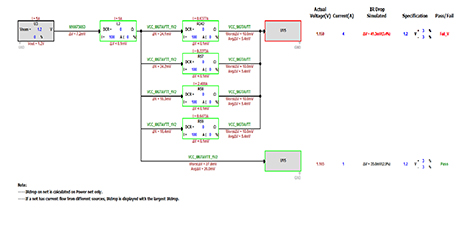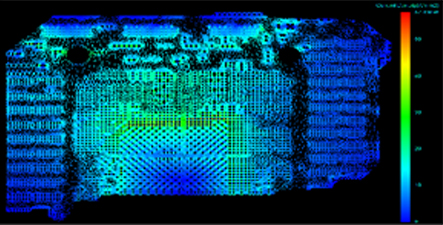PDN Impedance Analysis

-Impedance analysis of power supply network

-Noise and ripple analysis of power supply network

-Capacitance scheme optimization; capacitance design guidance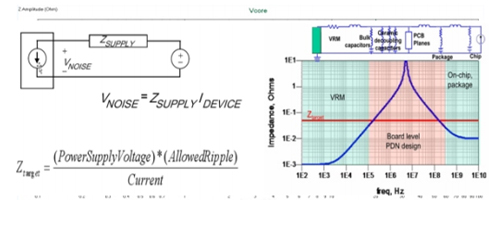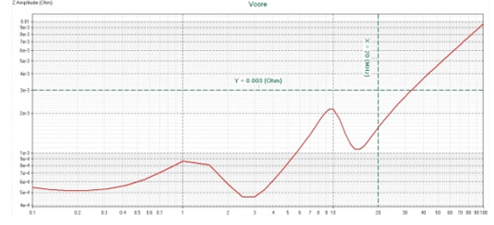Plane Resonance Analysis

-The plane resonance simulation analysis based on EMC requirements

-The risk assessment and design optimization of plane resonance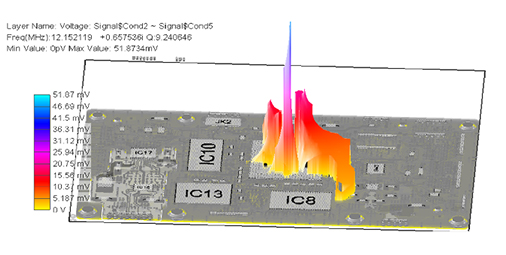Electrothermal Collaborative Simulation

- Synergistic analysis of static Joule heat, conductor conductivity and device power consumption

- Simulation of temperature distribution of single board and PCB design optimization